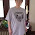## Thursday, December 31, 2020

### New cipher... Fibonacci

"Nasdaq" = 314 (Fibonacci)
"crypto currencies" = 661 (Fibonacci) 121st prime
"Bitcoin" = 461 (Fibonacci) 89th prime
"Bitcoin crypto currency" = 1063 (Fibonacci)178th prime
"crypto currency" = 602 (Fibonacci)
"United States Dollar" = 840 (Fibonacci)
"Dollar" = 470 (Fibonacci)
"Federal Reserve" = 309 (Fibonacci)
"Federal Reserve Note" = 704 (Fibonacci)
"Digital US Dollar" = 741 (Fibonacci) 38th Triangular
"Digital Currency" = 561 (Fibonacci) 33rd Triangular
"Bill and Melinda Gates Foundation" = 2087 (Fibonacci) 315th prime
"racism" = 325 (Fibonacci)25th triangular
"Lord" = 325 (Fibonacci)
"race" = 42 (Fibonacci)
"white" = 76 (Fibonacci)
"black" = 237 (Fibonacci)
"race riots" = 288 (Fibonacci)
"covid" = 188 (Fibonacci)
"sick" = 146 (Fibonacci)
"plague" = 260 (Fibonacci)
"pandemic" = 600 (Fibonacci)
"Bible" = 185 (Fibonacci)
"Book of Revelation" = 1148 (Fibonacci)
"end of days" = 419 (Fibonacci)81st prime
"Satan" = 269 (Fibonacci)
"Devil" = 191 (Fibonacci)

Found some interesting stuff on the Pope
"Pope Francis" = 660 (Fibonacci)
"coronavirus" = 660 (Fibonacci)
"Jorge Mario Bergoglio" = 1229 (Fibonacci)
201st prime

"Papa Francesco" = 630 (Fibonacci) 630+036=666

"Francis" = 333 (Fibonacci)
Nashville ATT building address was 333. Jeffery Epstein died on the 333rd day of the Jewish year. Text to Biden number was 30330 = 333. Pipe burst signal in Alanta zipcode was 30303. CNN headquarters zipcode 30303.

I've been seeing a lot of 333's lately maybe that's why!

"Vatican" = 289 (Fibonacci) sq rt 17 (1717)
"Jesuits" = 157 (Fibonacci) 37th prime
"Jesuit" = 136 (Fibonacci)

"Mark of Beast" = 550 (Fibonacci)

"US President" = 466 (Fibonacci) the 466th prime number is 3313

"March 13th 2020" = 333 (Fibonacci)
"March 13th 2013" = 335 (Fibonacci)

"Caesar" = 64 (Fibonacci)
"Julius Caesar" = 334 (Fibonacci)

"Sin" = 288 (Fibonacci)
"Man of Sin" = 907 (Fibonacci) 155th prime
"Godless" = 351 (Fibonacci) 26th Triangular
"false light" = 404 (Fibonacci)
"sinful" = 448 (Fibonacci)
"Genesis" = 332 (Fibonacci)
"Book of Genesis" = 862 (Fibonacci)
"Behold a pale horse" = 783 (Fibonacci)
"Behold a white horse" = 620 (Fibonacci)

"Elizabeth" = 225 (Fibonacci)
"Queen Elizabeth" = 531 (Fibonacci) 531+135=666
"Queen" = 306 (Fibonacci)
"King" = 369 (Fibonacci)
"Long Live The King" = 1130 (Fibonacci)
"Long Live The Queen" = 1067 (Fibonacci)
"Queen of England" = 1090 (Fibonacci)
"England" = 632 (Fibonacci)
"1600 Pennsylvania" = 1007 (Fibonacci)
"London" = 901 (Fibonacci)

"City of London" = 1103 (Fibonacci) 185th prime
"City of London England" = 1735 (Fibonacci) in duo 1007
"1600 Pennsylvania" = 1007 (Fibonacci)
"Rome" = 416 (Fibonacci)
"Bear Market" = 416 (Fibonacci)

"Putin" = 377 (Fibonacci) 14th Fibonacci
"Russia" = 119 (Fibonacci)
"Magog" = 404 (Fibonacci)
"Moscow" = 547 (Fibonacci) 101st prime
"war" = 38 (Fibonacci)
"Russian US war" = 419 (Fibonacci)
"end of days" = 419 (Fibonacci)
"Vladimir Putin" = 865 (Fibonacci) Duo of 1229 / 1229 is 201st prime
"President of Russia" = 708 (Fibonacci)
"KGB" = 103 (Fibonacci)

"Doctor Anthony Fauci" = 1039 (Fibonacci)
"Fauci" = 53 (Fibonacci)
"Georgetown" = 607 (Fibonacci) 111th prime

Revelation 8:11 and Wormwood seem to be coronavirus to me... 811, 577, 126, 141

"ritual blood sacrifice" = 811 (Fibonacci)141st prime In Duo: 577
"blood sacrifice" = 577 (Fibonacci) 106th prime
"sacrifice" = 141 (Fibonacci) 811 is the 141st prime number

"Wormwood Star" = 777 (Fibonacci)
"Wormwood" = 708 (Fibonacci)

"human sacrifice" = 637 (Fibonacci)

"Revelation 8:11" = 628 (Fibonacci)
"The Mark of the Beast" = 628 (Fibonacci)In Duo: 444

"And the name of the star is called Wormwood" = 2070 (Fibonacci)

1.That Fibonacci cypher looks awesome Joan. Can you share where to find the calculator for that cypher?
Thank you.

1.It's in the place where the Chaldean is in the "other" cipher section. If it doesn't come up for you clear your browser and it will.

http://www.gematrinator.com/calculator/index.php

2.Thank you very much for letting me know but unfortunately I still can't find it. Not to worry!
Love the Covid 188 & US President 466!